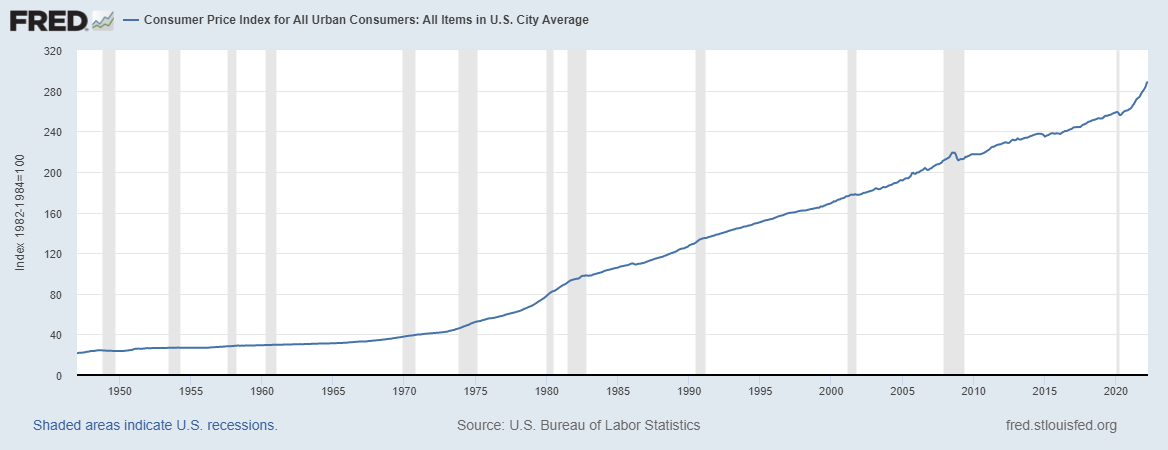# Why Central Banks have relied for too long on biased CPI Index methodologies, that have constantly misplaced price stability

May 14, 2022Views: 783

Similar methodologies in calculating Inflation & inflation % changes are too simplistic. These are methodologies based on index formulas that in the end calculate the sum of weighted % changes and are cumulative. The FRED CPI INDEX chart explains, quite well, the quasi exponential growth of the CPI Index, by design of its Summation % changes methodology. That fails to understand Price Inflation changes even at times of low & moderate price increases when Inflation in % tends to Central Banks 2.0% benchmark. Thereby, Monetary Policies have been misplaced.

The methodology design to measure Inflation requires observing supply & demand market data, with commodities prices, while also measuring Demand Elasticity and Inelasticity (-q’p/q), to then approximate Price levels for goods. Indeed, Statistical Multivariate methodology with Variance, Covariance and correlation, of goods prices % changes. Multivariate regression allows for determining how far Theta, Variance of certain parameters fluctuate (sigma/sqrt(n)) standard errors from the 2.0% inflation price stability, thereby combining those variables with the Central Banks money market interest rate tool, in understanding & modelling, going forward, the appropriate interest rate levels. This CPI Index chart started with 21.48 points in January 1947, to April 2022 with CPI 288.663 points.

## CPI INDEX Variance explains the Inflationary spirals of exponential growth of prices

Var= 660450% thousand, Standard Deviation=81286% thousand. So the Average CPI Index value, with this methodology, should be 114.99= 115 points, basically, the CPI INDEX INFLATED 173.66 points, that in \$dollar economic terms it’s way more than 8.5% CPI measured in 2022.

In fact, starting with 21.48 points to 288.663 points the CPI Index Inflated 13.44 times, INFLATED 1344% thousand. The mode value of this dataset = 29.84.

## Consumer Price Index for All Urban Consumers: Purchasing Power of the Consumer Dollar in U.S. City Average 1947-2022 series.

The USDollar and U.S. citizens have constantly lost Purchasing Power, while the economy Inflated away, between economic growth periods and recessions.